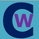1514 просмотров
1514
Updated and fixed version of DFC.
- fixed bugs
- added permanent setting for direction
- added color change for terminal and breakout bars
- added smart timings for line of 100% retracement
```// Created by PolarSolar 09 May 2016
// Updated: 09 May 2016

study(title="Donchian Fibo Channels v2", shorttitle="DFC2", overlay=true)
length = input(21, minval=8)
prz = input(0.618, title="PRZ level",minval=0.236, maxval=0.886, step=0.001)
dir = input(true, title="Uptrend?")
lo = lowest(round(dir?length*(1/prz):length))
hi = highest(round(dir?length:length*(1/prz)))
range = abs(hi-lo)
// calculate levels
r236 = dir>0?hi-(range*0.236):lo+(range*0.236)
r382 = dir>0?hi-(range*0.382):lo+(range*0.382)
r500 = avg(hi,lo)// basis
r618 = dir>0?hi-(range*0.618):lo+(range*0.618)
r707 = dir>0?hi-(range*0.707):lo+(range*0.707)
r786 = dir>0?hi-(range*0.786):lo+(range*0.786)
r886 = dir>0?hi-(range*0.886):lo+(range*0.886)
przl = dir>0?hi-(range*prz):lo+(range*prz)

plot(lo,    color=gray,     title="MIN", linewidth=2)
plot(hi,    color=gray,     title="MAX", linewidth=2)
plot(przl,  color=#EEE8AA,  title="PRZ", linewidth=2, trackprice=true) // special
plot(r236,  color=#BA55D3,  title=".236") // violet
plot(r382,  color=#4169E1,  title=".382") // blue
plot(r500,  color=#00BFFF,  title=".500") // light blue
plot(r618,  color=#32CD32,  title=".618") // green
plot(r707,  color=#FFD700,  title=".707") // yellow
plot(r786,  color=#FF8C00,  title=".786") // orange
plot(r886,  color=red,      title=".886") // red
// rebound
barcolor(dir==true  and  low<przl and close>przl and close>przl and close>przl?#7FFF00:na,offset=-1) // go up from PRZ
barcolor(dir==false and high>przl and close<przl and close<przl and close<przl?#FF1493:na,offset=-1)  // go dn from PRZ
// break
barcolor(dir>0 and close<przl?#DC143C:na,offset=-1) // PRZ breaked down
barcolor(dir==0 and close>przl?#32CD32:na,offset=-1)  // PRZ breaked up
```Wicked piece of coding!! Nice!! Great indicator. Learnt much from you. Thanks. :))
Ответить